Home > CCA2 > Chapter 7 > Lesson 7.2.3 > Problem7-149

7-149.
1.Compute the value of each expression without using a calculator. Homework Help ✎

1. log(8) + log(125)

2. log25(125)

3.log(25) + log(20)

4. 7log7(12)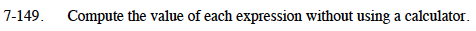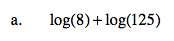Use the Product Property of Logs: log(N) + log(M) = log(NM).

log(8 · 125)

Remember you are working in log base 10.

log10(1000)

3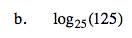Let the expression equal x then convert to exponential form.

25x = 125

Rewrite each side of the equation as a power of 5. The exponents are equal.

52x = 53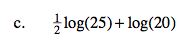Apply the Power Property of Logs to the first logarithm and simplify.

$\log (25)^{1/2} + \log (20)$

log(5) + log(20)

Use the Product Property of Logs, as in part (a).

$b^{\text{log}_b(N)} = N$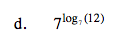Remember the property that